# 3D打印机静力温度流速误差分析研究Analysis and Study of Static Temperature Velocity of 3D Printer

DOI: 10.12677/MET.2018.72016, PDF, HTML, XML, 下载: 739  浏览: 1,361  科研立项经费支持

Abstract: The 3D printer is a precision machining equipment. The force of the structure of the body, the change of temperature, the change of the flow velocity of the material and so on will have a great influence on the machining precision. In view of the influence of load, temperature difference and velocity, the deformation of the 3D printer’s body will be caused, and the phenomenon of machining error will be analyzed in detail. In the process of design, the CAD and finite element model of the 3D printer extrusion mechanism, the worktable are established. The maximum point of the body deformation is found through the force deformation, the fluid analysis and the temperature change analysis of the model, and the visualization of the coupling field and the internal fluid in the temperature field of the extruder structure is converted to the visualization, which facilitates the adjustment and optimization of the design model. On the basis of the analysis of the data obtained from the operation analysis, the design of the whole machine body is perfected and optimized, which provides a scientific and effective method and necessary theoretical basis for the optimization design of the 3D printer.

1. 引言

3D打印机的加工方式以低速静态为主，随着制造技术高速发展，对设备的要求越来越高。为了提升3D打印机的加工精度，对3D打印机的整体结构进行分析，控制和减少力、温度、流体三耦合对3D打印机的影响越来越重要。由于通常采用带、螺纹进行部件的连接与传动，3D打印机加工零件误差是由3D打印机打印机部件连接处的误差及部件本身的弯曲变形造成的。因此在设计3D打印机整机结构的过程中，主要分析力、温度、材料流速三方面耦合，从而可以最大限度的控制和降低力、温差及材料流速三方面对打印机结构的影响，提高加工精度  。

2. 3D打印机整机结构示意图的建立

3D打印机(型号：LocorBot-Family，机器尺寸：(400 × 431 × 376) mm，加工尺寸：(205 × 160 × 140) mm，重量为：10.3 kg)整机结构主要由底板、工作台、X向带传动机构、Y向带传动机构、Z向螺旋传动机构、挤出机构六部分组成，如图1所示为结构示意图及实物图  。

3. 挤出机构及工作台的有限元分析

3.1. 整机静力理论分析

3D打印机是龙门桥式结构，整机受力变形简图如图2所示。移动挤出机构6在光杆3上，通过同步带沿着X方向做横向运动，产生附加惯性力，使光杆弯曲及移动龙门产生偏转。挤出机构、光杆3作用在Y方向传动机构上，通过同步带沿着Y方向做纵向运动，惯性力使挤出机构弯曲及移动龙门产生偏转；工作台通过丝杆在Z向移动，工件重量及工作台重量使工作台产生弯曲变形如图3所示；由3D打印机几何尺寸及材料属性可得整机分布载荷及集中载荷计算结果如下M1：1) 42步进电机重量为0.5 Kg；2) 喉管与喷嘴等其他零件总重为0.3 Kg；3) 光杠承受的载荷为2.4 kg；4) 加热块和丝材质计算大约为12 g；工作台载荷计算结果M2如下：1) 工作台重量1.5 Kg；2) 工件重量0.5 kg  。

3.2. 整机有限元模型的建立

3.3. 整机有限元静力分析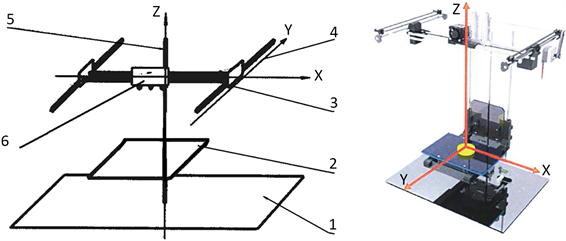1 底板；2 工作台；3 X方向带传动；4 Y方向带传动；5 Z方向螺旋传动；6 挤出机构

Figure 1. The structure diagram and the real figure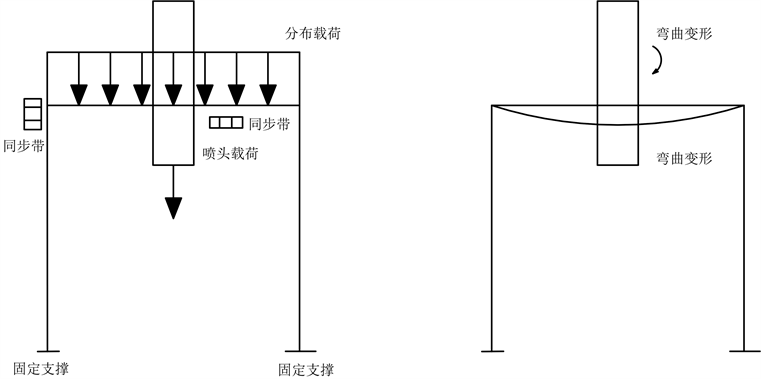Figure 2. The schematic diagram of static deformation of movable gantry bridge

Z向上由于受载是丝杠，载荷计算结果如下M3主要有：1) 工作台自身的重量；2) 成型工件重量；总受力载荷为21.1 N，方向垂直向下，通过ANSYS有限元分析，位移最大位置是工作台最边沿，这也和实际情况相符合，最大位移量0.00185 mm，变形百分比5 × 10−6%，整体形变属于微观形变，在正常范围，不会对机构运动造成影响。

4. 挤出机构泵流体速度场与温度场分析

4.1. 挤出机构三维模型建立

4.2. 挤出泵流体数学模型建立

${\zeta }_{ij}=-\rho {\delta }_{ij}+\lambda {\delta }_{ij}\nabla .u+2u{u}_{ij}$ (式1)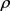——第二黏度；将方程式1带入到动量微分方程式中，得纳维·斯托克斯方程：

$\rho \frac{\text{d}{\mu }_{i}}{\text{d}t}=\rho {f}_{i}-\frac{\partial }{\partial {x}_{j}}\left(p+\frac{2}{3}\mu \nabla \mu \right)+\frac{\partial }{\partial {x}_{j}}\left[u\frac{\partial {\mu }_{i}}{\partial {x}_{i}}+\frac{\partial {\mu }_{i}}{\partial {x}_{j}}\right]$ (式2)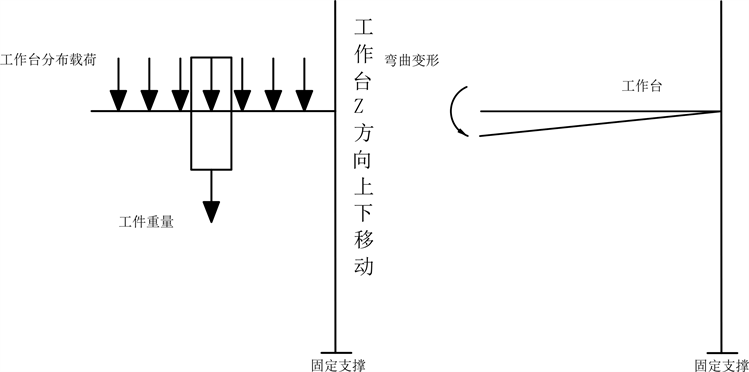Figure 3. The workbench static deformation diagram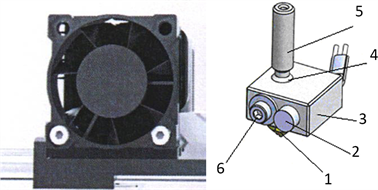1 喷嘴；2 加热棒；3 加热铝块：4 功U热腔；5 喉管；6 坝9温电偶

Figure 4. Extruder physical and CAD model

K方程：

$\frac{Dk}{Dt}=-\stackrel{¯}{{{u}^{\prime }}_{i}{{u}^{\prime }}_{l}}\frac{\stackrel{¯}{\partial {u}_{i}}}{\partial {x}_{j}}-\frac{\partial }{\partial {x}_{j}}\left(\stackrel{¯}{{k}^{\prime }{{u}^{\prime }}_{l}}+\frac{1}{\rho }\stackrel{¯}{{p}^{\prime }{{u}^{\prime }}_{l}}-\nu \frac{\partial k}{\partial {x}_{l}}\right)-\epsilon$ (式3)

$\stackrel{¯}{{k}^{\prime }{{u}^{\prime }}_{l}}+\frac{1}{\rho }\stackrel{¯}{{p}^{\prime }{{u}^{\prime }}_{l}}$ ——动能k与压能 $\frac{{p}^{\prime }}{p}$ 的脉动迁移变化率

$\nu \frac{\partial k}{\partial {x}_{l}}$ ——扩散项

$\epsilon$ ——粘性耗散系数 $\epsilon =\stackrel{¯}{\nu \frac{\partial {{u}^{\prime }}_{I}}{\partial {x}_{j}}}\frac{\stackrel{¯}{\partial {{u}^{\prime }}_{I}}}{\partial {x}_{i}}$

4.3. 求解器边界条件设置

a、因为该问题包含流体的运动和温度场分析，两者互相影响，所以选择运动、温度耦合求解。

b、根据温度实际情况，整个温度分析过程包含加热和保温两阶段。

c、定义各材料属性并参数。

d、设定环境边界选项，将大气压设置为标准大气压，温度设置为室内温度，设置重力加速度数值与方向。

e、设置求解器极限值。挤出泵从室内温度开始加热，温度达到要求的工作温度，系统控制散热器和加热棒对挤出泵实施保温处理。参照挤出泵温度变化过程，设置泵体温度下限值为室内温度(300 k)，上限值为工作时温度(500 k)。

4.4. 挤出泵温度分布云图分析Figure 5. Initial state temperature distribution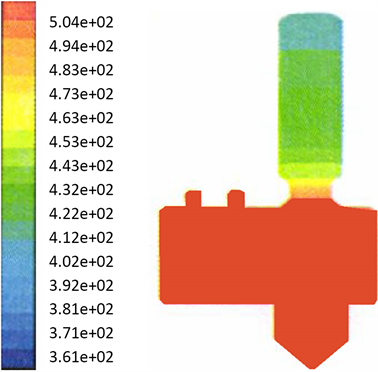Figure 6. Distribution of working state temperature

4.5. 挤出泵速度场分析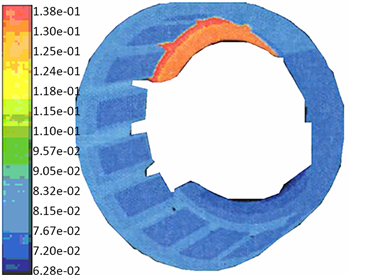Figure 7. The initial velocity distribution in the inner basin of the extrusion mechanism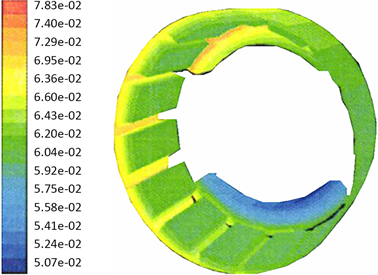Figure 8. The flow velocity distribution state of the Working state in the extrusion mechanism

5. 结论

2016年江苏省淮安市基金项目支持(HAP201680)。

  万巍. 熔融沉积型快速成型制作中的问题分析、研究和解决[J]. 河北工业科技, 2012, 29(6): 376-380.  吴春, 陈军, 湛彪. 滑片累性能试验台设计[J]. 石油化工设备, 2010, 39(1): 14-16.  张斌, 徐兵, 杨华勇, 等. 基于虚拟样机技术的数字式柱塞累控制特性研究[J]. 浙江大学学报(工学版), 2010, 44(1): 1. 7.  崔万瑞 等. 3D打印机体系结构研究[J]. 计算机盘软件与应用, 2014(16): 127-128.  张应迁, 唐克伦. 基于ANSYS的FLUENT前处理[J]. 机械工程师, 2012(6): 51-52.  姬宜朋, 张沛, 王丽. 注射过程喷嘴内物料温度和压力的模拟与分折[J]. 工程塑料应用, 2004, 32(9): 23-26.  章梓雄. 粘性流体力学[M]. 北京: 清华大学出版社, 1998.